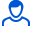Content: teorver_5591.doc (28.50 KB)

Positive responses: 0
Negative responses: 0

Refunds: 05591. x̅=16.8, n=25, σ=5. Based on the sample size n=25, the arithmetic mean x̅v=16.8 was found. Assuming that X is a normally distributed random variable, find the confidence interval that, with reliability γ=0.99, covers the unknown mathematical expectation a if the general standard deviation σ=5
Detailed solution. Decorated in Microsoft Word 2003 (Quest decided to use the formula editor)
No feedback yet